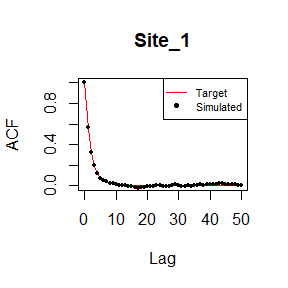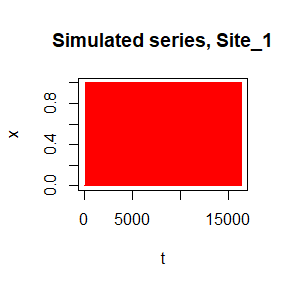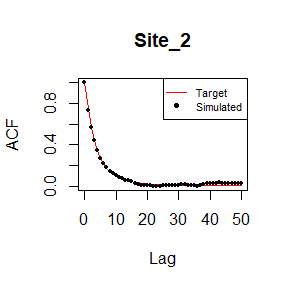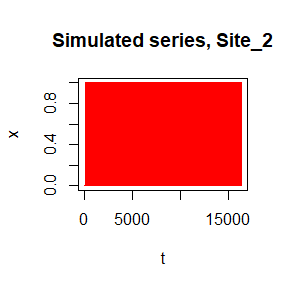# 1 Load the required R packages

library(anySim)
library(DEoptim)
library(pracma)
library(Rsolnp)
library(matrixcalc)
library(psych)

# 2 Stochastic simulation of univariate processes

## 2.1 Stationary processes

### 2.1.1 The ARTA(p) model

This model uses an appropriately parameterised AR(p) to simulate an auxiliary Gaussian process (Gp) to establish the target correlation structure. In the final step, the Gp realisation is mapped to the actual domain through the ICDF of the target distribution.

#### 2.1.1.1 Continuous marginal distributions

Simulation of a process with gamma marginal distribution, with shape=1, and scale=1. In this case, the target autocorrelation structure is from an fGn process (i.e., Hurst) with H=0.7.

# Define the dependence structure (autocorrelation coefficients)
ACF=acsHurst(H=0.7, lag=500, var=1)

# Define the marginal distribution
fx='qgamma'
pfx=list(shape=1, scale=1)

# Estimate the parameters of ARTA(p) model
ARTApar=EstARTAp(ACF=ACF, maxlag=0, dist=fx, params=pfx,
NatafIntMethod ='GH', NoEval=9, polydeg=0)

# Simulate the process
Sim=SimARTAp(ARTApar = ARTApar, burn = 1000, steps = 10^5, stand = 0)

# Compare the target and simulated autocorrelation structure
plot(0:50, ACF[1:51],type = "l",col="red",xlab = "Lag",ylab = "ACF",main=NULL)
points(0:50,as.vector(acf(Sim$X,lag.max = 50,plot = FALSE)$acf),col="black",pch=19,cex=0.5)
legend("topright",c("Target","Simulated"),col=c("red",'black'),
lwd=c(1,NA), lty=c(1,NA),pch=c(NA,19),box.lty=1,cex=0.7)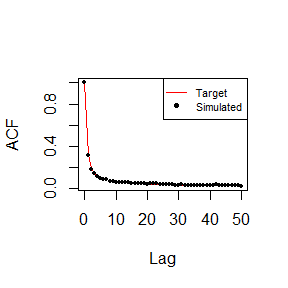# Compare target and simulated marginal distribution
plot(sort(Sim$X[1:3000]),ppoints(Sim$X[1:3000]),pch=19,cex=0.5,
col="black",main=NULL,xlab="x",ylab=bquote(P(italic(underline(x)<=plain(x)))))
lines(qgamma(seq(0,0.999,by = 0.001),scale=1, shape=1),seq(0,0.999,by = 0.001),col='red',cex=0.5)
legend("bottomright",c("Target","Simulated"),col=c("red",'black'),
lwd=c(1,NA), lty=c(1,NA),pch=c(NA,19),box.lty=1,cex=0.7)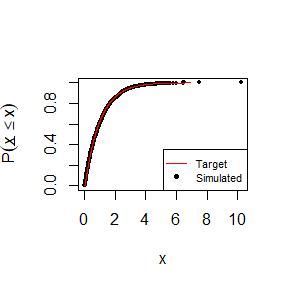# Plot the series
plot(Sim$X[1:1000],type='l',col='red',ylab="x",main="Simulated series",xlab="t")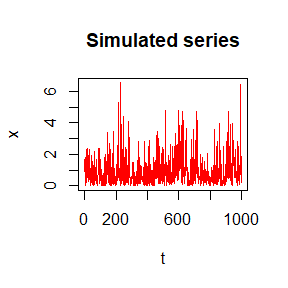Simulation of a process with Beta marginal distribution, with shape=2, and shape2=10. In this case, the target autocorrelation structure is from an fGn process (i.e., Hurst) with H=0.7. # Define the dependence structure (autocorrelation coefficients) ACF=acsHurst(H=0.7, lag=500, var=1) # Define the marginal distribution fx='qbeta' pfx=list(shape1=2,shape2=10) # Estimate the parameters of ARTA(p) model ARTApar=EstARTAp(ACF=ACF, maxlag=0, dist=fx, params=pfx, NatafIntMethod ='GH', NoEval=9, polydeg=0) # Simulate the process Sim=SimARTAp(ARTApar = ARTApar, burn = 1000, steps = 10^5, stand = 0) # Compare the target and simulated autocorrelation structure plot(0:50, ACF[1:51],type = "l",col="red",xlab = "Lag",ylab = "ACF",main=NULL) points(0:50,as.vector(acf(Sim$X,lag.max = 50,plot = FALSE)$acf),col="black",pch=19,cex=0.5) legend("topright",c("Target","Simulated"),col=c("red",'black'), lwd=c(1,NA), lty=c(1,NA),pch=c(NA,19),box.lty=1,cex=0.7)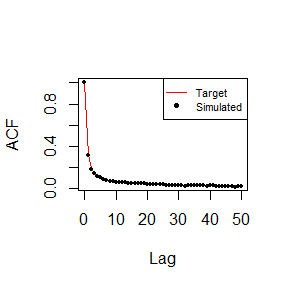# Compare target and simulated marginal distribution plot(sort(Sim$X[1:3000]),ppoints(Sim$X[1:3000]),pch=19,cex=0.5, col="black",main=NULL,xlab="x",ylab=bquote(P(italic(underline(x)<=plain(x))))) lines(qbeta(seq(0,0.999,by = 0.001),shape1=2,shape2=10),seq(0,0.999,by = 0.001),col='red',cex=0.5) legend("bottomright",c("Target","Simulated"),col=c("red",'black'), lwd=c(1,NA), lty=c(1,NA),pch=c(NA,19),box.lty=1,cex=0.7)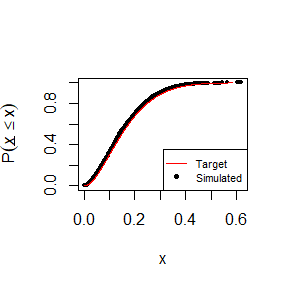# Plot the series plot(Sim$X[1:1000],type='l',col='red',ylab="x",main="Simulated series",xlab="t")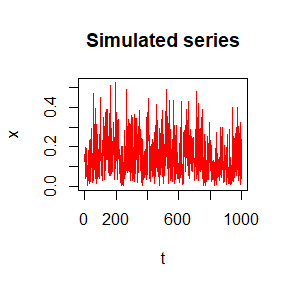# acf(Sim$X) # lines(0:(length(ACF)-1), ACF) # plot(Sim$X[1:1000], type='l', col='red')

Simulation of a process with Burr type-XII marginal distribution, with shape=2, shape2=2, and scale=1. In this case, the target autocorrelation structure is from an fGn process (i.e., Hurst) with H=0.7.

# Define the dependence structure (autocorrelation coefficients)
ACF=acsHurst(H=0.7, lag=500, var=1)

# Define the marginal distribution
# load the actuar library which contains the Burr type-XII distribution
library(actuar)
fx='qburr'
pfx=list(shape1=2,shape2=2,scale=1)

# Estimate the parameters of ARTA(p) model
ARTApar=EstARTAp(ACF=ACF, maxlag=0, dist=fx, params=pfx,
NatafIntMethod ='GH', NoEval=9, polydeg=0)

# Simulate the process
Sim=SimARTAp(ARTApar = ARTApar, burn = 1000, steps = 10^5, stand = 0)

# Compare the target and simulated autocorrelation structure
plot(0:50, ACF[1:51],type = "l",col="red",xlab = "Lag",ylab = "ACF",main=NULL)
points(0:50,as.vector(acf(Sim$X,lag.max = 50,plot = FALSE)$acf),col="black",pch=19,cex=0.5)
legend("topright",c("Target","Simulated"),col=c("red",'black'),
lwd=c(1,NA), lty=c(1,NA),pch=c(NA,19),box.lty=1,cex=0.7)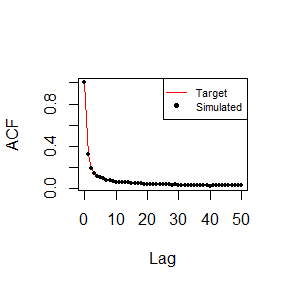# Compare target and simulated marginal distribution
plot(sort(Sim$X[1:3000]),ppoints(Sim$X[1:3000]),pch=19,cex=0.5,
col="black",main=NULL,xlab="x",ylab=bquote(P(italic(underline(x)<=plain(x)))))
lines(qburr(seq(0,0.999,by = 0.001),shape1=2,shape2=2,scale=1),
seq(0,0.999,by = 0.001),col='red',cex=0.5)
legend("bottomright",c("Target","Simulated"),col=c("red",'black'),
lwd=c(1,NA), lty=c(1,NA),pch=c(NA,19),box.lty=1,cex=0.7)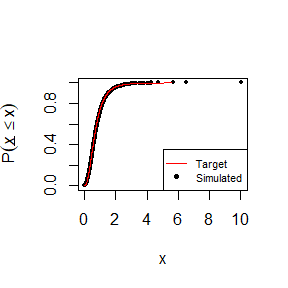# Plot the series
plot(Sim$X[1:1000],type='l',col='red',ylab="x",main="Simulated series",xlab="t")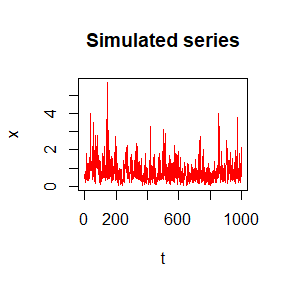# acf(Sim$X)
# lines(0:(length(ACF)-1), ACF)
# plot(Sim$X[1:1000], type='l', col='red') #### 2.1.1.2 Discrete marginal distributions Simulation of a process with Binomial marginal distribution, with size=1, and prob=0.2. In this case, the target autocorrelation structure is from an fGn process (i.e., Hurst) with H=0.7. # Define the dependence structure (autocorrelation coefficients) ACF=acsHurst(H=0.7, lag=500, var=1) # Define the marginal distribution fx='qbinom' pfx=list(size=1,prob=0.2) # Estimate the parameters of ARTA(p) model ARTApar=EstARTAp(ACF=ACF, maxlag=0, dist=fx, params=pfx, NatafIntMethod ='Int', NoEval=9, polydeg=0) # Simulate the process Sim=SimARTAp(ARTApar = ARTApar, burn = 1000, steps = 10^5, stand = 0) # Compare the target and simulated autocorrelation structure plot(0:50, ACF[1:51],type = "l",col="red",xlab = "Lag",ylab = "ACF",main=NULL) points(0:50,as.vector(acf(Sim$X,lag.max = 50,plot = FALSE)$acf),col="black",pch=19,cex=0.5) legend("topright",c("Target","Simulated"),col=c("red",'black'), lwd=c(1,NA), lty=c(1,NA),pch=c(NA,19),box.lty=1,cex=0.7)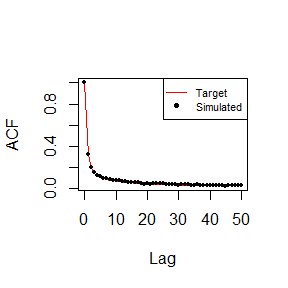# Compare target and simulated marginal distribution p0<-length(which(Sim$X==0))/length(Sim$X);print(paste0("Empirical probability zero is: ",p0)) #>  "Empirical probability zero is: 0.79247" p1<-length(which(Sim$X==1))/length(Sim$X);print(paste0("Empirical probability one is: ",p1)) #>  "Empirical probability one is: 0.20753" # Plot the series plot(Sim$X[1:1000],type='l',col='red',ylab="x",main="Simulated series",xlab="t")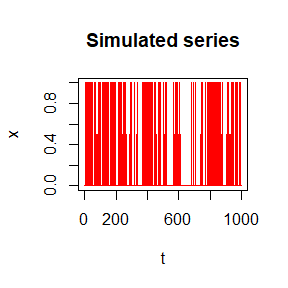Simulation of a process with Poisson marginal distribution, with lamda=3. In this case, the target autocorrelation structure is from an fGn process (i.e., Hurst) with H=0.7.

# Define the dependence structure (autocorrelation coefficients)
ACF=acsHurst(H=0.7, lag=500, var=1)

# Define the marginal distribution
fx='qpois'
pfx=list(lambda=3)

# Estimate the parameters of ARTA(p) model
ARTApar=EstARTAp(ACF=ACF, maxlag=0, dist=fx, params=pfx,
NatafIntMethod ='Int', NoEval=9, polydeg=0)

# Simulate the process
Sim=SimARTAp(ARTApar = ARTApar, burn = 1000, steps = 10^5, stand = 0)

# Compare the target and simulated autocorrelation structure
plot(0:50, ACF[1:51],type = "l",col="red",xlab = "Lag",ylab = "ACF",main=NULL)
points(0:50,as.vector(acf(Sim$X,lag.max = 50,plot = FALSE)$acf),col="black",pch=19,cex=0.5)
legend("topright",c("Target","Simulated"),col=c("red",'black'),
lwd=c(1,NA), lty=c(1,NA),pch=c(NA,19),box.lty=1,cex=0.7)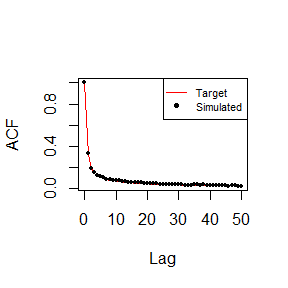# Compare target and simulated marginal distribution
plot(sort(Sim$X),ppoints(Sim$X),pch=19,cex=0.5,type="l",
col="black",main=NULL,xlab="x",ylab=bquote(P(italic(underline(x)<=plain(x)))))
lines(qpois(seq(0,0.999,by = 0.001),lambda=3),seq(0,0.999,by = 0.001),col='red',cex=0.5)
legend("bottomright",c("Target","Simulated"),col=c("red",'black'),
lwd=c(1,NA), lty=c(1,NA),pch=c(NA,19),box.lty=1,cex=0.7)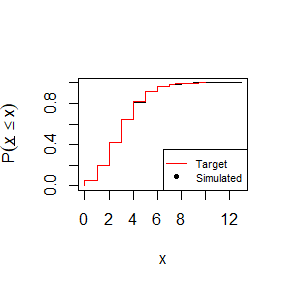# Plot the series
plot(Sim$X[1:1000],type='l',col='red',ylab="x",main="Simulated series",xlab="t")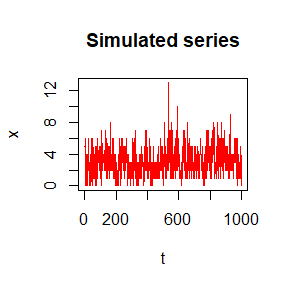# acf(Sim$X)
# lines(0:(length(ACF)-1), ACF)
# plot(Sim$X[1:1000], type='l', col='red') #### 2.1.1.3 zero-inflated marginal distributions Simulation of a process with zero-inflated (mixed) marginal distribution, with p0=0.8 (probability of zero values, discrete part) and Gamma distribution (nonzero values, continuous part) with shape=1, and scale=1. In this case, the target autocorrelation structure is from an fGn process (i.e., Hurst) with H=0.7. # Define the dependence structure (autocorrelation coefficients) ACF=acsHurst(H=0.7, lag=500, var=1) # Define the marginal distribution fx='qmixed' p0=0.8 pfx=list(Distr=qgamma, p0=0.8, shape=1, scale=1) # Estimate the parameters of ARTA(p) model ARTApar=EstARTAp(ACF=ACF, maxlag=0, dist=fx, params=pfx, NatafIntMethod ='GH',NoEval=9, polydeg=0) # Simulate the process Sim=SimARTAp(ARTApar = ARTApar, burn = 1000, steps = 10^5, stand = 0) # Estimate probability of zero values of the simulated series SimNonZero<-Sim$X[Sim$X>0] PdrSim<-round(mean(Sim$X<=0),3);PdrSim
#>  0.8

# Compare the target and simulated autocorrelation structure
plot(0:50, ACF[1:51],type = "l",col="red",xlab = "Lag",ylab = "ACF",main=NULL)
points(0:50,as.vector(acf(Sim$X,lag.max = 50,plot = FALSE)$acf),col="black",pch=19,cex=0.5)
legend("topright",c("Target","Simulated"),col=c("red",'black'),
lwd=c(1,NA), lty=c(1,NA),pch=c(NA,19),box.lty=1,cex=0.7)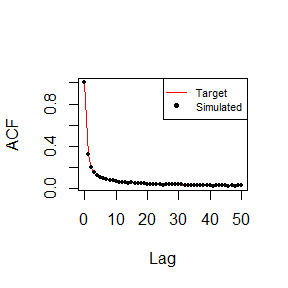# Compare target and simulated marginal distribution of the nonzero values
plot(sort(SimNonZero[1:3000]),ppoints(SimNonZero[1:3000]),pch=19,cex=0.5,
col="black",main=NULL,xlab="x",ylab=bquote(P(italic(underline(x)<=plain(x)))))
lines(qgamma(seq(0,0.999,by = 0.001),scale=1, shape=1),seq(0,0.999,by = 0.001),col='red',cex=0.5)
legend("bottomright",c("Target","Simulated"),col=c("red",'black'),
lwd=c(1,NA), lty=c(1,NA),pch=c(NA,19),box.lty=1,cex=0.7)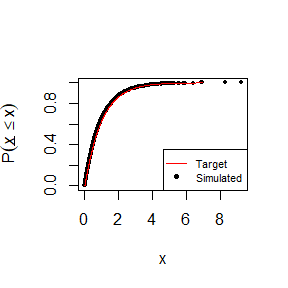# Plot the series
plot(Sim$X[1:1000],type='l',col='red',ylab="x",main="Simulated series",xlab="t")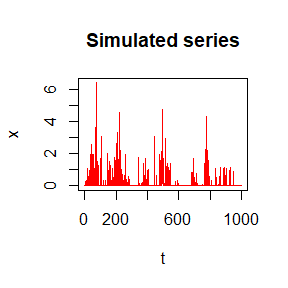# acf(Sim$X)
# lines(0:(length(ACF)-1), ACF)
# plot(Sim$X[1:1000], type='l', col='red') ### 2.1.2 The nARTA(1) model This model uses the sum of n, appropriately parameterised, AR(1) models to simulate an auxiliary Gaussian process (Gp) to establish the target correlation structure. In the final step, the Gp realisation is mapped to the actual domain through the ICDF of the target distribution. #### 2.1.2.1 Continuous marginal distributions Simulation of a process with gamma marginal distribution, with shape=1, and scale=1. In this case, the target autocorrelation structure is givern from an CAS ACS with b=2 and k=0.5. # Define the dependence structure (autocorrelation coefficients) ACF=acsCAS(param=c(2, 0.5),lag=500,var=1) # Define the marginal distribution fx='qgamma' pfx=list(shape=1,scale=1) # Estimate the parameters of n-ARTA(1) model nAR1par=EstnAR1(ACF=ACF, Ar1Num = 4, dist=fx, params=pfx, NatafIntMethod ='GH', NoEval=9, polydeg=0) #> #> Iter: 1 fn: 0.0005953 Pars: 0.47470 0.88969 0.98173 0.99860 0.39507 0.33060 0.16819 0.10614 #> Iter: 2 fn: 0.0005953 Pars: 0.47470 0.88969 0.98173 0.99860 0.39507 0.33060 0.16819 0.10614 #> solnp--> Completed in 2 iterations # Simulate the process Sim=SimnAR1(nAR1param = nAR1par, steps = 10^5) # Compare the target and simulated autocorrelation structure plot(0:50, ACF[1:51],type = "l",col="red",xlab = "Lag",ylab = "ACF",main=NULL) points(0:50,as.vector(acf(Sim$X,lag.max = 50,plot = FALSE)$acf),col="black",pch=19,cex=0.5) legend("topright",c("Target","Simulated"),col=c("red",'black'), lwd=c(1,NA), lty=c(1,NA),pch=c(NA,19),box.lty=1,cex=0.7)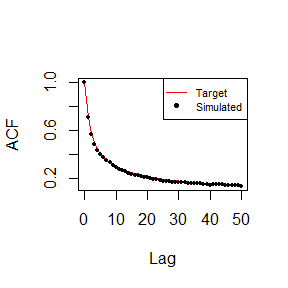# Compare target and simulated marginal distribution plot(sort(Sim$X[1:3000]),ppoints(Sim$X[1:3000]),pch=19,cex=0.5, col="black",main=NULL,xlab="x",ylab=bquote(P(italic(underline(x)<=plain(x))))) lines(qgamma(seq(0,0.999,by = 0.001),scale=1, shape=1),seq(0,0.999,by = 0.001),col='red',cex=0.5) legend("bottomright",c("Target","Simulated"),col=c("red",'black'), lwd=c(1,NA), lty=c(1,NA),pch=c(NA,19),box.lty=1,cex=0.7)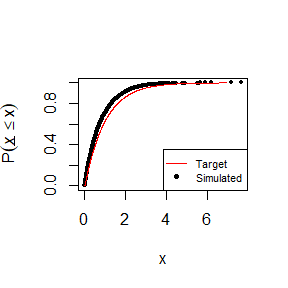# Plot the series plot(Sim$X[1:1000],type='l',col='red',ylab="x",main="Simulated series",xlab="t")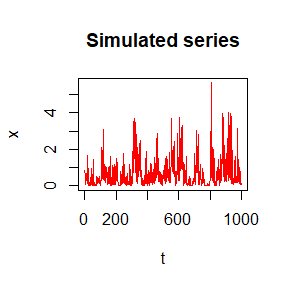# acf(Sim$X) # lines(0:(length(ACF)-1), ACF) # plot(Sim$X[1:1000], type='l', col='red')

The simulation procedure for discrete, or zero-inflated processes, is the same as in the case of ARTA(p) model.

### 2.1.3 The SMARTA model

This model uses an appropriately parameterised SMA(q) model to simulate an auxiliary Gaussian process (Gp) to establish the target correlation structure. In the final step, the Gp realisation is mapped to the actual domain through the ICDF of the target distribution.

#### 2.1.3.1 Continuous marginal distributions

Simulation of a process with gamma marginal distribution, with shape=1, and scale=1. In this case, the target autocorrelation structure is from an CAS ACS with b=2 and k=0.5.

# Define the dependence structure (autocorrelation coefficients)
ACF=acsCAS(param = c(2, 0.5), lag=512, var=1)
ACFs=list(ACF)

# Define the marginal distribution
fx='qgamma'
pfx=list(shape=1, scale=1)
pfxs=list(pfx)

# Estimate the parameters of SMARTA model
SMARTApar=EstSMARTA(dist = fx, params = pfxs, ACFs = ACFs, Cmat = NULL, DecoMethod ='cor.smooth', FFTLag = 512,
NatafIntMethod ='GH', NoEval=9, polydeg=0)

# Simulate the process
Sim=SimSMARTA(SMARTApar = SMARTApar, steps = 10^5, SMALAG = 512)

# Compare the target and simulated autocorrelation structure
plot(0:50, ACF[1:51],type = "l",col="red",xlab = "Lag",ylab = "ACF",main=NULL)
points(0:50,as.vector(acf(Sim$X,lag.max = 50,plot = FALSE)$acf),col="black",pch=19,cex=0.5)
legend("topright",c("Target","Simulated"),col=c("red",'black'),
lwd=c(1,NA), lty=c(1,NA),pch=c(NA,19),box.lty=1,cex=0.7)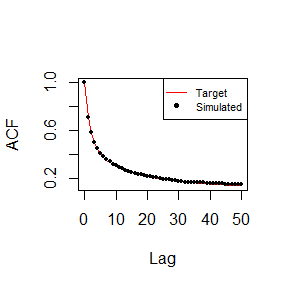# Compare target and simulated marginal distribution
plot(sort(Sim$X[1:3000]),ppoints(Sim$X[1:3000]),pch=19,cex=0.5,
col="black",main=NULL,xlab="x",ylab=bquote(P(italic(underline(x)<=plain(x)))))
lines(qgamma(seq(0,0.999,by = 0.001),scale=1, shape=1),seq(0,0.999,by = 0.001),col='red',cex=0.5)
legend("bottomright",c("Target","Simulated"),col=c("red",'black'),
lwd=c(1,NA), lty=c(1,NA),pch=c(NA,19),box.lty=1,cex=0.7)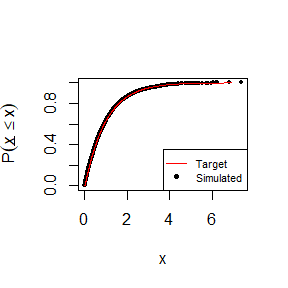# Plot the series
plot(Sim$X[1:1000],type='l',col='red',ylab="x",main="Simulated series",xlab="t")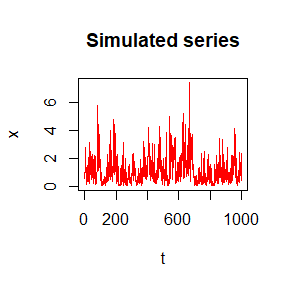# acf(Sim$X)
# lines(0:(length(ACF)-1), ACF)
# plot(Sim$X[1:1000], type='l', col='red') The simulation procedure for discrete, or zero-inflated processes, is the same as in the case of ARTA(p) model. ## 2.2 Stochastic simulation of cyclostationary processes ### 2.2.1 The SPARTA model This model uses an appropriately parameterised PAR(1) model to simulate a cyclostationary auxiliary Gaussian process (Gp) to establish the target season-to-season correlation structure. In the final step, the cyclostationary Gp realisation is mapped on season-wise basis to the actual domain through the ICDF of the target distributions. Simulation of cyclostationary process with 12 seasons. #### 2.2.1.1 zero-inflated marginal distributions # Define the number of seasons NumOfSeasons=12 # Define the season-to-season correlations rtarget<-c(0.7,0.6,0.3,0.5,0.6,0.7,0.5,0.6,0.7,0.8,0.7,0.6) # Define the marginal distributions for each season FXs<-rep('qmixed',NumOfSeasons) PFXs<-vector("list",NumOfSeasons) PFXs[]=list(p0=0.4, Distr=qgamma, scale=1, shape=1) PFXs[]=list(p0=0.5, Distr=qweibull, scale=1, shape=1) PFXs[]=list(p0=0.2, Distr=qgamma, scale=1, shape=0.8) PFXs[]=list(p0=0.1, Distr=qlnorm, meanlog=1, sdlog=1) PFXs[]=list(p0=0.0, Distr=qgamma, scale=1, shape=1) PFXs[]=list(p0=0.4, Distr=qweibull, scale=1, shape=1) PFXs[]=list(p0=0.5, Distr=qgamma, scale=1, shape=0.8) PFXs[]=list(p0=0.3, Distr=qlnorm, meanlog=1, sdlog=1) PFXs[]=list(p0=0.2, Distr=qgamma, scale=1, shape=1) PFXs[]=list(p0=0.1, Distr=qweibull, scale=1, shape=1) PFXs[]=list(p0=0.4, Distr=qgamma, scale=1, shape=0.8) PFXs[]=list(p0=0.3, Distr=qlnorm, meanlog=1, sdlog=1) # Estimate the parameters of SPARTA model SPARTApar<-EstSPARTA(s2srtarget = rtarget, dist = FXs, params = PFXs, NatafIntMethod = 'GH', NoEval = 9, polydeg = 8, nodes=11) # Simulate the process Sim<-SimSPARTA(SPARTApar = SPARTApar, steps=100000, stand=0) # Estimate season-to-season correlations of the simulated series seasonCor<-round(s2scor(Sim$X),3);seasonCor
#>   0.705 0.596 0.297 0.500 0.591 0.698 0.499 0.598 0.696 0.800 0.699
#>  0.603

# Estimate probability of zero values of the simulated series for each season
seasonSimNonZero<-apply(Sim$X,2,function(x) x[x>0]) seasonPdrSim<-round(apply(Sim$X,2,function(x) mean(x<=0)),3);seasonPdrSim
#>  Season_1  Season_2  Season_3  Season_4  Season_5  Season_6  Season_7
#>     0.398     0.499     0.202     0.102     0.000     0.402     0.499
#>  Season_8  Season_9 Season_10 Season_11 Season_12
#>     0.300     0.199     0.100     0.400     0.300

# Compare the target and simulated season-to-season correlations
plot(rtarget,type = "l",col="red",xlab="Season",ylab="Lag-1",main=NULL)
points(seasonCor,col='black',pch=19,cex=0.7)
legend("bottomright",c("Target","Simulated"),col=c("red",'black'),
lwd=c(1,NA), lty=c(1,NA),pch=c(NA,19),box.lty=1,cex=0.7)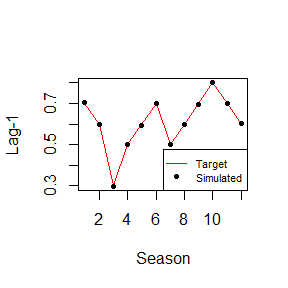# Compare target and simulated marginal distributions for indicative seasons
# Season 1
plot(sort(seasonSimNonZero$Season_1[1:3000]),ppoints(seasonSimNonZero$Season_1[1:3000]),
pch=19,cex=0.5,col="black",main="Season_1, Gamma",
xlab="x",ylab=bquote(P(italic(underline(x)<=plain(x)))))
lines(qgamma(seq(0,0.999,by = 0.001),scale=1, shape=1),seq(0,0.999,by = 0.001),col='red',cex=0.5)
legend("bottomright",c("Target","Simulated"),col=c("red",'black'),
lwd=c(1,NA), lty=c(1,NA),pch=c(NA,19),box.lty=1,cex=0.7)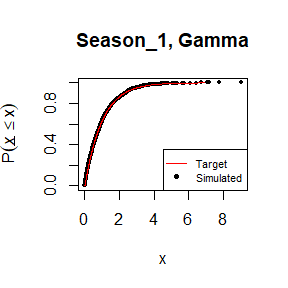# Season 6
plot(sort(seasonSimNonZero$Season_6[1:3000]),ppoints(seasonSimNonZero$Season_6[1:3000]),
pch=19,cex=0.5,col="black",main="Season_6, Weibull",
xlab="x",ylab=bquote(P(italic(underline(x)<=plain(x)))))
lines(qweibull(seq(0,0.999,by = 0.001),scale=1, shape=1),seq(0,0.999,by = 0.001),col='red',cex=0.5)
legend("bottomright",c("Target","Simulated"),col=c("red",'black'),
lwd=c(1,NA), lty=c(1,NA),pch=c(NA,19),box.lty=1,cex=0.7)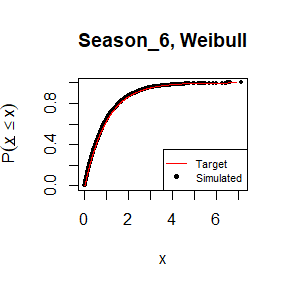# Season 12
plot(sort(seasonSimNonZero$Season_12[1:3000]),ppoints(seasonSimNonZero$Season_12[1:3000]),
pch=19,cex=0.5,col="black",main="Season_12, Lognormal",
xlab="x",ylab=bquote(P(italic(underline(x)<=plain(x)))))
lines(qlnorm(seq(0,0.999,by = 0.001),meanlog=1, sdlog=1),seq(0,0.999,by = 0.001),col='red',cex=0.5)
legend("bottomright",c("Target","Simulated"),col=c("red",'black'),
lwd=c(1,NA), lty=c(1,NA),pch=c(NA,19),box.lty=1,cex=0.7)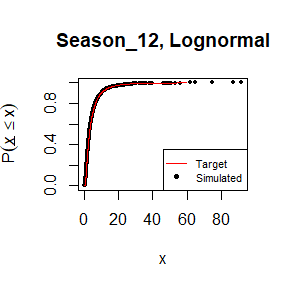# Plot the series
plot(Sim$X[1:1000],type='l',col='red',ylab="x",main="Simulated series",xlab="t")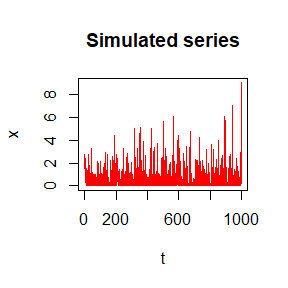The simulation procedure for discrete, or zero-inflated processes, is the same as in the case of ARTA(p) model. # 3 Stochastic simulation of multivariate processes ## 3.1 Stationary processes ### 3.1.1 The SMARTA model This model uses an appropriately parameterised multivariate SMA(q) model to simulate an auxiliary Gaussian process (Gp) to establish the target correlation structure. In the final step, the multivariate Gp realisation is mapped to the actual domain through the ICDF of the target distributions. #### 3.1.1.1 Zero-inflated marginal distributions Simulation of a bivariate process. # Setup simulation experiment LAG=2^6 FFTLag=2^7 SMALAG=2^6 steps=2^14 # Define the marginal distribution of the two processes PFXs=list() FXs=c('qmixed','qmixed') # Gamma distribution: Gamma(shape=2, rate=1) PFXs[]=list(Distr=qgamma, p0=0.9, shape=1, scale=1) # Weibull distribution: Weibull(shape=1, scale=2) PFXs[]=list(Distr=qweibull, p0=0.85, shape=1, scale=2) # Define the dependence structure (autocorrelation coefficients) of the two processes ACFs=list() ACFs[]=acsCAS(param = c(0.1, 0.6), lag = LAG) ACFs[]=acsCAS(param = c(0.2, 0.3), lag = LAG) # Define the lag-0 cross-correlation coefficient of the two processes Cmat=matrix(c(1,0.6,0.6,1), ncol=2, nrow=2) # Estimate the parameters of the multivariate SMARTA model SMAparam=EstSMARTA(dist = FXs, params = PFXs, ACFs = ACFs, Cmat = Cmat, DecoMethod = 'cor.smooth',FFTLag = FFTLag, NatafIntMethod = 'GH', NoEval = 9, polydeg = 8) # Simulate the multivariate SMARTA process Sim=SimSMARTA(SMARTApar = SMAparam, steps = steps, SMALAG = SMALAG) # Estimate probability of zero values of the simulated series SimNonZero<-apply(Sim$X,2,function(x) x[x>0])
PdrSim<-round(apply(Sim$X,2,function(x) mean(x<=0)),3);PdrSim #>  0.903 0.853 # Some basic plots for the two processes for (i in 1:2) { # Compare the target and simulated autocorrelation structure plot(0:50, ACFs[[i]][1:51],type = "l",col="red",xlab = "Lag",ylab = "ACF",main=paste0("Site_",i)) points(0:50,as.vector(acf(Sim$X[,i],lag.max = 50,plot = FALSE)$acf),col="black",pch=19,cex=0.5) legend("topright",c("Target","Simulated"),col=c("red",'black'), lwd=c(1,NA), lty=c(1,NA),pch=c(NA,19),box.lty=1,cex=0.7) # Compare target and simulated marginal distribution plot(sort(SimNonZero[[i]]),ppoints(SimNonZero[[i]]),pch=19,cex=0.5, col="black",main=paste0("Site_",i),xlab="x",ylab=bquote(P(italic(underline(x)<=plain(x))))) pfxtemp<-PFXs[[i]] pfxtemp$p0<-0
pfxtemp$p<-seq(0,0.999,by = 0.001) xtemp<-do.call(FXs[[i]],pfxtemp) lines(xtemp,pfxtemp$p,col='red',cex=0.5)
legend("bottomright",c("Target","Simulated"),col=c("red",'black'),
lwd=c(1,NA), lty=c(1,NA),pch=c(NA,19),box.lty=1,cex=0.7)

# Plot the series
plot(Sim$X[,i],type='l',col='red',ylab="x",main=paste0("Simulated series, Site_",i),xlab="t") }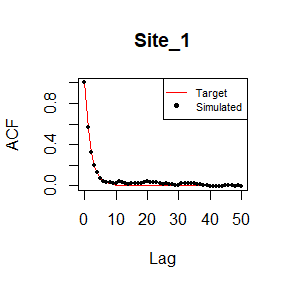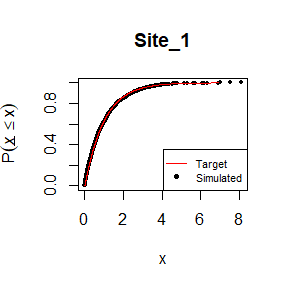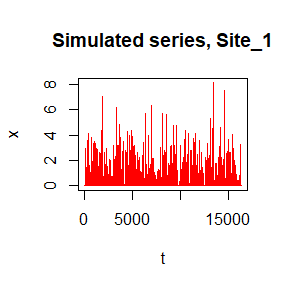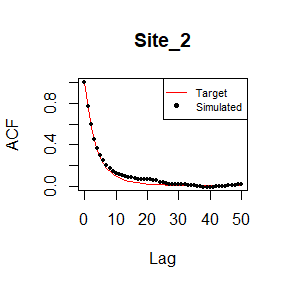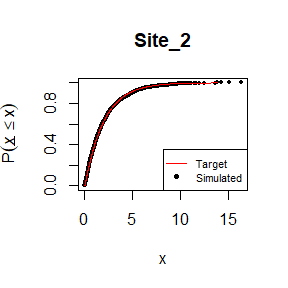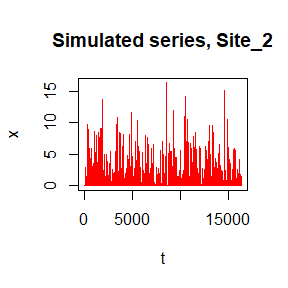#### 3.1.1.2 Discrete marginal distributions Simulation of a bivariate process with Binomial marginal distributions. # Define the marginal distribution of the two processes PFXs=list() FXs=c('qbinom','qbinom') PFXs[]=list(size=1, prob=0.2)# Gamma distribution: Gamma(shape=2, rate=1) PFXs[]=list(size=1, prob=0.25) # Weibull distribution: Weibull(shape=1, scale=2) # Define the dependence structure (autocorrelation coefficients) of the two processes ACFs=list() ACFs[]=acsCAS(param = c(0.1, 0.6), lag = LAG) ACFs[]=acsCAS(param = c(0.2, 0.3), lag = LAG) # Define the lag-0 cross-correlation coefficient of the two processes Cmat=matrix(c(1,0.6,0.6,1), ncol=2, nrow=2) # Estimate the parameters of the multivariate SMARTA model SMAparam=EstSMARTA(dist = FXs, params = PFXs, ACFs = ACFs, Cmat = Cmat, DecoMethod = 'cor.smooth', FFTLag = FFTLag, NatafIntMethod = 'Int', NoEval = 9, polydeg = 8) # Simulate the multivariate SMARTA process Sim=SimSMARTA(SMARTApar = SMAparam, steps = steps, SMALAG = SMALAG) # Compare target and simulated marginal distribution p0<-p1<-c() for(i in 1:2){ p0<-round(c(p0,length(which(Sim$X[,i]==0))/length(Sim$X[,i])),3) p1<-round(c(p1,length(which(Sim$X[,i]==1))/length(Sim$X[,i])),3) } print(paste0("Empirical probability zero for the two processes are: ",p0," and ",p0)) #>  "Empirical probability zero for the two processes are: 0.794 and 0.744" print(paste0("Empirical probability one for the two processes are: ",p1," and ",p1)) #>  "Empirical probability one for the two processes are: 0.206 and 0.256" # Some basic plots for the two processes for (i in 1:2) { # Compare the target and simulated autocorrelation structure plot(0:50, ACFs[[i]][1:51],type = "l",col="red",xlab = "Lag",ylab = "ACF",main=paste0("Site_",i)) points(0:50,as.vector(acf(Sim$X[,i],lag.max = 50,plot = FALSE)$acf),col="black",pch=19,cex=0.5) legend("topright",c("Target","Simulated"),col=c("red",'black'), lwd=c(1,NA), lty=c(1,NA),pch=c(NA,19),box.lty=1,cex=0.7) # Plot the series plot(Sim$X[,i],type='l',col='red',ylab="x",main=paste0("Simulated series, Site_",i),xlab="t")
}